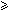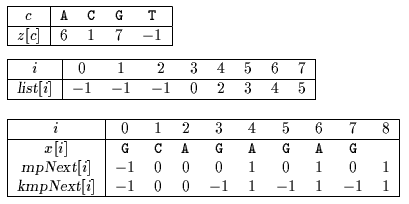Next: Alpha Skip Search algorithm Up: ESMAJ Prev: Skip Search algorithm

# KMP Skip Search algorithm

Main features
• improvement of the Skip Search algorithm;
• uses buckets of positions for each character of the alphabet;
• preprocessing phase in O(m+) time and space complexity;
• searching phase in O(n) time complexity.
Description

It is possible to make the Skip Search algorithm (see chapter Skip Search algorithm) linear using the two shift tables of Morris-Pratt (see chapter Morris and Pratt algorithm) and Knuth-Morris-Pratt (see chapter Knuth, Morris and Pratt algorithm).

For 1im, mpNext[i] is equal to the length of the longest border of x[0 .. i-1] and mpNext=-1.

For 1i < m, kmpNext[i] is equal to length of the longest border of x[0 .. i-1] followed by a character different from x[i], kmpNext=-1 and kmpNext[m]=m-per(x).

The lists in the buckets are explicitly stored in a table list.

The preprocessing phase of the KmpSkip Search algorithm is in O(m+) time and space complexity.

A general situation for an attempt during the searching phase is the following ((see figure 30.1):j is the current text position;x[i] = y[j];start = j-i is the possible starting position of an occurrence of x in y;wall is the rightmost scanned text position;x[0 .. wall-start-1] = y[start .. wall-1];Figure 30.1: General situation during the searching phase of the linear algorithm.

The comparisons are performed from left to right between x[wall-start .. m-1] and y[wall .. start+m-1] until a mismatch or a whole match occurs. Let kwall-start be the smallest integer such that x[ky[start+k] or k = m if an occurrence of x starts at position start in y.

Then wall takes the value of start+k.

After that the algorithm KmpSkip computes two shifts (two new starting positions): the first one according to the skip algorithm (see algorithm AdvanceSkip for details), this gives us a starting position skipStart, the second one according to the shift table of Knuth-Morris-Pratt, which gives us another starting position kmpStart.

Several cases can arise:skipStart < kmpStart then a shift according to the skip algorithm is applied which gives a new value for skipStart, and we have to compare again skipStart and kmpStart;kmpStart < skipStart < wall then a shift according to the shift table of Morris-Pratt is applied. This gives a new value for kmpStart. We have to compare again skipStart and kmpStart;skipStart = kmpStart then another attempt can be performed with start = skipStart;kmpStart < wall < skipStart then another attempt can be performed with start = skipStart.

The searching phase of the KmpSkip Search algorithm is in O(n) time.

The C code

The function preMp is given chapter Morris and Pratt algorithm and the function preKmp is given chapter Knuth, Morris and Pratt algorithm.

```int attempt(char *y, char *x, int m, int start, int wall) {
int k;

k = wall - start;
while (k < m && x[k] == y[k + start])
++k;
return(k);
}

void KMPSKIP(char *x, int m, char *y, int n) {
int i, j, k, kmpStart, per, start, wall;
int kmpNext[XSIZE], list[XSIZE], mpNext[XSIZE],
z[ASIZE];

/* Preprocessing */
preMp(x, m, mpNext);
preKmp(x, m, kmpNext);
memset(z, -1, ASIZE*sizeof(int));
memset(list, -1, m*sizeof(int));
z[x] = 0;
for (i = 1; i < m; ++i) {
list[i] = z[x[i]];
z[x[i]] = i;
}

/* Searching */
wall = 0;
per = m - kmpNext[m];
i = j = -1;
do {
j += m;
} while (j < n && z[y[j]] < 0);
if (j >= n)
return;
i = z[y[j]];
start = j - i;
while (start <= n - m) {
if (start > wall)
wall = start;
k = attempt(y, x, m, start, wall);
wall = start + k;
if (k == m) {
OUTPUT(start);
i -= per;
}
else
i = list[i];
if (i < 0) {
do {
j += m;
} while (j < n && z[y[j]] < 0);
if (j >= n)
return;
i = z[y[j]];
}
kmpStart = start + k - kmpNext[k];
k = kmpNext[k];
start = j - i;
while (start < kmpStart ||
(kmpStart < start && start < wall)) {
if (start < kmpStart) {
i = list[i];
if (i < 0) {
do {
j += m;
} while (j < n && z[y[j]] < 0);
if (j >= n)
return;
i = z[y[j]];
}
start = j - i;
}
else {
kmpStart += (k - mpNext[k]);
k = mpNext[k];
}
}
}
}

```
The example

Preprocessing phaseTables used by KMP Skip Search algorithm.

Searching phase

References
• CHARRAS C., LECROQ T., PEHOUSHEK J.D., 1998, A very fast string matching algorithm for small alphabets and long patterns, in Proceedings of the 9th Annual Symposium on Combinatorial Pattern Matching , M. Farach-Colton ed., Piscataway, New Jersey, Lecture Notes in Computer Science 1448, pp 55-64, Springer-Verlag, Berlin.Next: Alpha Skip Search algorithm Up: ESMAJ Prev: Skip Search algorithm

e-mails: {Christian.Charras, Thierry.Lecroq }@laposte.net
Tue Jan 14 15:03:31 MET 1997